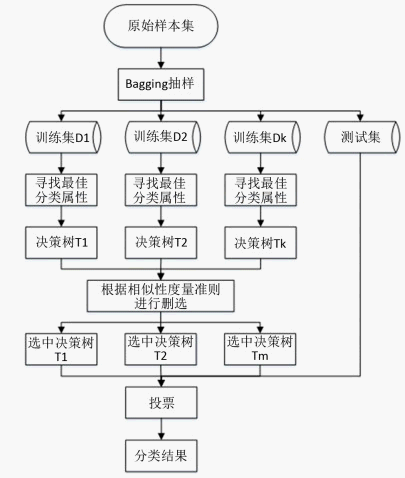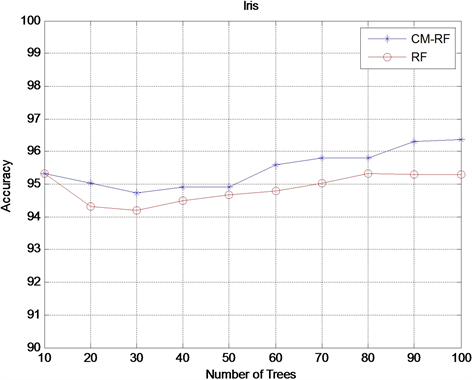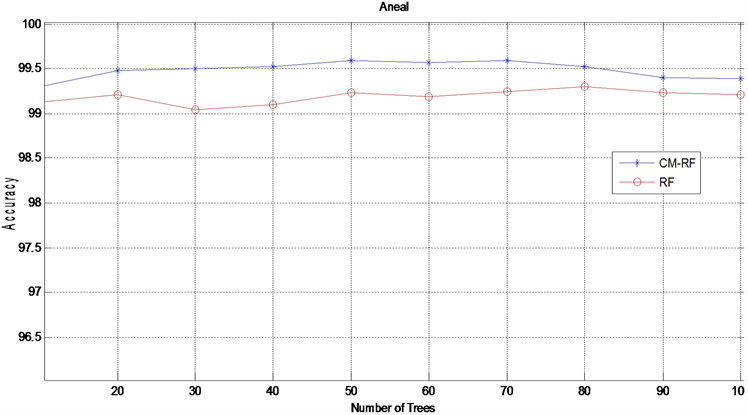# 用于无人机巡线的图像分类模型选择算法研究Research on Algorithm of Image Classification Model Selection for UAV Patrol

DOI: 10.12677/CSA.2020.109162, PDF, HTML, XML, 下载: 81  浏览: 240  科研立项经费支持

Abstract: As a classic classification algorithm, random forest algorithm is widely used and has high classification accuracy. However, in the process of classification, the classification performance of each decision tree and the difference between two decision trees are two important factors that affect the final classification effect. When some decision trees have similar misclassifications, and they are used in the final voting on the results of the decision tree, the final classification effect of the model will be reduced. Aiming at this problem, this paper proposes a method for measuring the similarity of decision trees based on confusion Matrix. This method takes into account the number of different categories of trees and the correct and incorrect classification, in order to select decision trees with weak similarity, and then remove the decision trees with poor classification results, and finally complete the model selection of random forest. Experimental results show that the method pro-posed in this paper has a higher average classification accuracy rate and higher stability in the three types of datasets.

1. 引言

2. 集成学习

${E}_{total}={E}_{bay}+\frac{1+\rho \left(N-1\right)}{N}{E}_{each}$ (1)

3. 随机森林算法

1) 从训练集中采用bootstrap法，即自助抽样法，有放回地抽取若干样本，作为一个训练子集。

2) 对于训练子集，从特征集中无放回地随机抽取若干特征，作为决策树的每个节点的分裂的依据。

3) 重复步骤1)和步骤2)，得到若干训练子集，并生产若干决策树，将决策树组合起来，形成随机森林。

4) 将测试集的样本输入随机森林中，让每个决策树对样本进行决策，得到结果后，采用投票方法对结果投票，得到样本的分类结果。

5) 重复步骤4)，直到测试集分类完成。

4. 随机森林模型选择

4.1. 本文提出的模型选择方法

4.1.1. 基于误差矩阵判断分类树相似性

$CN=\left[\begin{array}{ccc}c{n}_{11}& \cdots & c{n}_{1m}\\ ⋮& c{n}_{ii}& ⋮\\ c{n}_{m1}& ...& c{n}_{mm}\end{array}\right]$ (2)

$DC{N}^{\left(i,j\right)}=C{N}^{\left(i\right)}-C{N}^{\left(j\right)}=\left[\begin{array}{cccc}c{n}_{11}^{\left(i\right)}-c{n}_{11}^{\left(j\right)}& c{n}_{12}^{\left(i\right)}-c{n}_{12}^{\left(j\right)}& \cdots & c{n}_{1N}^{\left(i\right)}-c{n}_{1M}^{\left(j\right)}\\ c{n}_{21}^{\left(i\right)}-c{n}_{21}^{\left(j\right)}& c{n}_{22}^{\left(i\right)}-c{n}_{22}^{\left(j\right)}& \cdots & c{n}_{2N}^{\left(i\right)}-c{n}_{2M}^{\left(j\right)}\\ ⋮& ⋮& \ddots & ⋮\\ c{n}_{M1}^{\left(i\right)}-c{n}_{M1}^{\left(j\right)}& c{n}_{M2}^{\left(i\right)}-c{n}_{M2}^{\left(j\right)}& \cdots & c{n}_{MM}^{\left(i\right)}-c{n}_{MM}^{\left(j\right)}\end{array}\right]$ (3)

$dc{{n}^{\prime }}_{mn}=\frac{dc{n}_{mn}}{ma{x}_{m}}$ (4)

$ma{x}_{m}=ma{x}_{n}\left(dc{n}_{mn}\right)$ (5)

$r{f}_{ij}=\left\{\begin{array}{l}0,\text{\hspace{0.17em}}\text{\hspace{0.17em}}\left(i\ge j\right)\\ {‖DC{{N}^{\prime }}_{i,j}‖}_{F}=\sqrt{{\sum }_{m}^{M}{\sum }_{n}^{M}dc{{n}^{\prime }}_{mn}^{2}},\text{\hspace{0.17em}}\text{\hspace{0.17em}}\left(i (6)

$r{f}_{ij}$ 越小，则树i与树j的相似度越高，两个分类器对样本的分类结果越接近。Figure 1. Random forest selection model

4.1.2. 基于“删劣”策略选择模型

4.1.3. 模型选择算法描述

1：通过决策树对测试样本进行分类预测；

2：根据分类结果，为决策树创建误差矩阵 $CN$

3：创建相似度度量矩阵 ${R}_{F}$

4：for (( $i,j=1$ to l) & ( $i ))

5：令 ${m}_{ij}$${R}_{F}$ 中最小的非零元素

6：for ( ${m}_{ij} )

if (决策树i分类效果 $<\beta$ )

${R}_{F}$ 中的树i清除

${m}_{ij}=$ ${R}_{F}$ 中下一个最小非零元素

7：否则结束，未删除的决策树组成随机森林RF。

5. 实验与分析

5.1. 实验数据说明Table 1. Experimental data set taken from UCI

5.2. 实验结果与分析Figure 2. Accuracy comparison results on the Iris datasetFigure 3. Accuracy comparison results on the Breast-cancer datasetFigure 4. Accuracy comparison results on the Aneal dataset

6. 结束语

  Babar, B., Luppino, L.T., Boström, T. and Anfinsen, S.N. (2020) Random Forest Regression for Improved Mapping of Solar Irradiance at High Latitudes. Solar Energy, 198, 81-92. https://doi.org/10.1016/j.solener.2020.01.034  Li, J., Tian, Y., Zhu, Y., Zhou, T.S., Li, J., Ding, K.F. and Li, J.S. (2020) A Multicenter Random Forest Model for Effective Prognosis Prediction in Collaborative Clinical Research Network. Artificial Intelligence in Medicine, 103, Article ID: 101814. https://doi.org/10.1016/j.artmed.2020.101814  Hammou, B.A., Lahcen, A.A. and Mouline, S. (2019) An Effective Distributed Predictive Model with Matrix Factorization and Random Forest for Big Data Recommendation Systems. Expert Systems with Applications, 137, 253-265. https://doi.org/10.1016/j.eswa.2019.06.046  Çifçi, M.A., Ertugrul, D.Ç. and Elçi, A. (2016) A Search Service for Food Consumption Mobile Applications via Hadoop and MapReduce Technology. 2016 IEEE 40th Annual Comput-er Software and Applications Conference (COMPSAC), Atlanta, 10-14 June 2016, 77-82. https://doi.org/10.1109/COMPSAC.2016.35  刘迎春, 陈梅玲. 流式大数据下随机森林方法及应用[J]. 西北工业大学学报, 2015, 33(6): 1055-1061.  Yousfi, S. and Chiadmi, D. (2015) Big Data-as-a-Service Solution for Building Graph Social Networks. 2015 International Conference on Cloud Technologies and Applications (CloudTech), Marrakech, 2-4 June 2015, 1-6. https://doi.org/10.1109/CloudTech.2015.7337009  韩伟, 张学庆, 陈旸. 基于MapReduce的图像分类方法[J]. 计算机应用, 2014, 34(6): 1600-1603.  Rajagopalan, M.R. and Vellaipandiyan, S. (2013) Big Data Framework for National E-Governance Plan. 2013 11th International Conference on ICT and Knowledge Engineering (ICT&KE), Bangkok, 20-22 November 2013, 1-5. https://doi.org/10.1109/ICTKE.2013.6756283  孙悦, 袁健. 基于Spark的改进随机森林算法[J]. 电子科技, 2019, 32(4): 60-63+67.  Gall, J., Yao, A., Razavi, N., Cool, L.V. and Lempitsky, V. (2011) Hough Forests for Ob-ject Detection, Tracking, and Action Recognition. IEEE Transactions on Pattern Analysis and Machine Intelligence, 33, 2188-2202. https://doi.org/10.1109/TPAMI.2011.70  Gall, J. and Lempitsky, V. (2009) Class-Specific Hough Forests for Object Detection. 2009 IEEE Computer Society Conference on Computer Vision and Pattern Recognition, Miami, 20-25 June 2009, 1022-1029. https://doi.org/10.1109/CVPR.2009.5206740  Ishwaran, H., Kogalur, U.B., Xi C. and Minn, A.J. (2011) Random Survival Forests for High-Dimensional Data. Statistical Analysis and Data Mining, 4, 115-132. https://doi.org/10.1002/sam.10103  谢晓龙, 叶笑冬, 董亚明. 梯度提升随机森林模型及其在日前出清电价预测中的应用[J]. 计算机应用与软件, 2018, 35(9): 327-333.  魏正韬. 基于非平衡数据的随机森林算法研究[D]: [硕士学位论文]. 西安: 西安电子科技大学, 2017.  王诚, 高蕊. 基于特征约减的随机森林改进算法研究[J/OL]. 计算机技术展, 2020, 30(3): 40-45.  雍凯. 随机森林的特征选择和模型优化算法研究[D]: [硕士学位论文]. 哈尔滨: 哈尔滨工业大学, 2008.  毕凯, 王晓丹, 姚旭, 等. 一种基于Bagging和混淆矩阵的自适应选择性集成[J]. 电子学报, 2014(4): 711-716.  Tumer, K. and Ghosh, J. (1996) Error Correlation and Error Reduc-tion in Ensemble Classifiers. Connection Science, 8, 385-340. https://doi.org/10.1080/095400996116839  Faria, F.A., Dos Santos, J.A., Sarkar, S., et al. (2013) Classifier Selection Based on the Correlation of Diversity Measures: When Fewer Is More. 2013 XXVI Conference on Graphics, Patterns and Images, Arequipa, 5-8 August 2013, 16-23. https://doi.org/10.1109/SIBGRAPI.2013.12  Shi, H.L., Ferguson, D., Beagley, J. and Huyck, M. (2008) Work in Progress—Improving Interrater Agreement Used to Measure Learning Outcomes. 2008 38th Annual Frontiers in Ed-ucation Conference, Saratoga Springs, 22-25 October 2008, F2B-7-F2B-8. https://doi.org/10.1109/FIE.2008.4720398  Löfström, T., Johansson, U. and Boström, H. (2008) On the Use of Accuracy and Diversity Measures for Evaluating and Selecting Ensembles of Classifiers. 2008 7th International Con-ference on Machine Learning and Applications (ICLMLA), San Diego, 11-13 December 2008, 127-132. https://doi.org/10.1109/ICMLA.2008.102  乔少杰, 唐常杰, 陈瑜, 等. 基于树编辑距离的层次聚类算法[J]. 计算机科学与探索, 2007, 1(3): 282-292.  谢元澄, 杨静宇. 删除最差基学习器来层次修剪Bagging集成[J]. 计算机研究与发展, 2009, 46(2): 261-267.